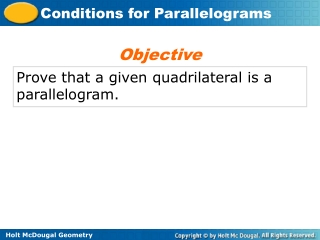DownloadDownload PresentationProve that a given quadrilateral is a parallelogram.

# Prove that a given quadrilateral is a parallelogram.

Download Presentation## Prove that a given quadrilateral is a parallelogram.

- - - - - - - - - - - - - - - - - - - - - - - - - - - E N D - - - - - - - - - - - - - - - - - - - - - - - - - - -
##### Presentation Transcript

1. Objective Prove that a given quadrilateral is a parallelogram.

2. You can use the given information about a figure to decide which condition is best to apply.

3. The two theorems below can also be used to show that a given quadrilateral is a parallelogram.

4. Example 1A: Verifying Figures are Parallelograms Show that JKLM is a parallelogram for a = 3 and b = 9. Step 1 Find JK and LM. Given LM = 10a + 4 JK = 15a – 11 Substitute and simplify. LM = 10(3)+ 4 = 34 JK = 15(3) – 11 = 34

5. Example 1B: Verifying Figures are Parallelograms Show that PQRS is a parallelogram for x = 10 and y = 6.5. mQ = (6y + 7)° Given Substitute 6.5 for y and simplify. mQ = [(6(6.5) + 7)]° = 46° mS = (8y – 6)° Given Substitute 6.5 for y and simplify. mS = [(8(6.5) – 6)]° = 46° mR = (15x – 16)° Given Substitute 10 for x and simplify. mR = [(15(10) – 16)]° = 134°

6. Example 1B Continued Since 46° + 134° = 180°, R is supplementary to both Q and S. PQRS is a parallelogram by Theorem 6-3-4.

7. PQ = RS = 16.8, so Therefore, Check It Out! Example 1 Show that PQRS is a parallelogram for a = 2.4 and b = 9. mQ = 74°, and mR = 106°, so Q and R are supplementary. So one pair of opposite sides of PQRS are || and . By Theorem 6-3-1, PQRS is a parallelogram.

8. Example 2A: Applying Conditions for Parallelograms Determine if the quadrilateral must be a parallelogram. Justify your answer. Yes. The 73° angle is supplementary to both its corresponding angles. By Theorem 6-3-4, the quadrilateral is a parallelogram.

9. Example 2B: Applying Conditions for Parallelograms Determine if the quadrilateral must be a parallelogram. Justify your answer. No. One pair of opposite angles are congruent. The other pair is not. The conditions for a parallelogram are not met.

10. Check It Out! Example 2a Determine if the quadrilateral must be a parallelogram. Justify your answer. Yes The diagonal of the quadrilateral forms 2 triangles. Two angles of one triangle are congruent to two angles of the other triangle, so the third pair of angles are congruent by the Third Angles Theorem. So both pairs of opposite angles of the quadrilateral are congruent . By Theorem 6-3-3, the quadrilateral is a parallelogram.

11. Check It Out! Example 2b Determine if each quadrilateral must be a parallelogram. Justify your answer. No. Two pairs of consective sides are congruent. None of the sets of conditions for a parallelogram are met.